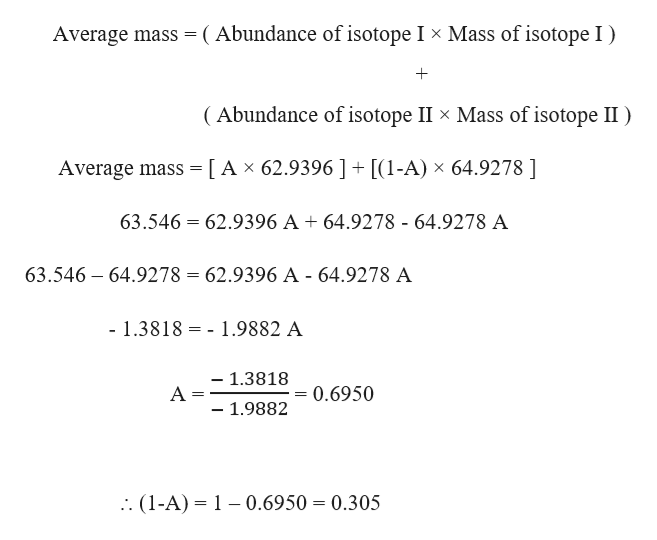# Copper has two naturally occurring isotopes, 63Cu (isotopic mass 62.9396 amu) and 65Cu (isotopic mass 64.9278 amu). If copper has an atomic mass of 63.546 amu, what is the percent abundance of 65Cu?

Question

Copper has two naturally occurring isotopes, 63Cu (isotopic mass 62.9396 amu) and 65Cu (isotopic mass 64.9278 amu). If copper has an atomic mass of 63.546 amu, what is the percent abundance of 65Cu?

check_circle

Step 1

Given,

Mass of 63Cu (isotope I) = 62.9396 amu

Mass of 65Cu (isotope II) = 64.9278 amu

Atomic mass of Cu (average mass) = 63.546 amu

Let ‘A’ be the abundance of 63Cu (isotope I) then, (1-A) will be the abundance of 65Cu (isotope II).

The average mass of Cu is expressed as:help_outlineImage TranscriptioncloseAverage mass (Abundance of isotope I x Mass of isotope I (Abundance of isotope II x Mass of isotope II) Average mass [Ax 62.9396 ] + [(1-A) x 64.9278] = 63.546 62.9396 A + 64.9278 - 64.9278 A 63.546 64.9278 62.9396 A - 64.9278 A - 1.3818 -1.9882 A - 1.3818 A = - 1.9882 0.6950 . (1-A) 1- 0.6950 0.305 fullscreen
Step 2

The percent abundance of the Cu isotopes will be:

A =...

### Want to see the full answer?

See Solution

#### Want to see this answer and more?

Solutions are written by subject experts who are available 24/7. Questions are typically answered within 1 hour.*

See Solution
*Response times may vary by subject and question.
Tagged in

### General Chemistry